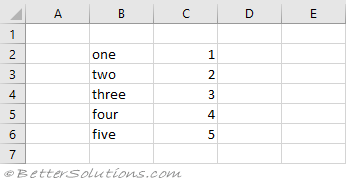# VBA Code

Use the Application.WorksheetFunction. object to call one of the built-in Excel worksheet functions.
Use the VBA. object to call one of the built-in VBA functions.

### Application.WorksheetFunction.Sum

The SUM function returns the total value of the numbers in a list or cell range.It is possible to leave off the "Application." prefix as this is a global member.

`Call MsgBox(Application.WorksheetFunction.Sum(1, 2, 3, 4, 5)) = 15 Call MsgBox(WorksheetFunction.Sum(1, 2, 3, 4, 5)) = 15 `

You can pass an Excel range into this function.

`' the result must be declared as a Variant and not a String.Dim lookup_result As Variant Dim myRange As Excel.Range Set myRange = Range("C2:C6") lookup_result = Application.WorksheetFunction.Sum(myRange) Call MsgBox(lookup_result) = 15 `

You can even pass an array into this function.

`Dim lookup_result As Variant Dim myArray As Variant myArray = Array(1, 2, 3, 4, 5) lookup_result = Application.WorksheetFunction.Sum(myArray) Call MsgBox(lookup_result) = 15 `

### Application.Sum

This is not a VBA function, it is just a confusing syntax for calling the built-in Excel worksheet functions.

`Call MsgBox(Application.Sum(1, 2, 3, 4, 5)) = 15 `

This syntax is a shortcut to "Application.WorksheetFunction.Sum".
If you use this "abbreviated" or "reduced" syntax you will not see any intellisense.
Although, the intellisense you do get from using the WorksheetFunction. object isn't great, its better than nothing.
Avoid using this notation because to most people this looks like it could be a genuine VBA function.

### Application.WorksheetFunction.VLookup

The VLOOKUP function returns the value in the same row after finding a matching value in the first column.`Call MsgBox(Application.WorksheetFunction.VLookup("three", Range("B2:C6"), 2, False)) = 3 `

You can pass an Excel range into this function.

`' the result must be declared as a Variant and not a String.Dim lookup_result As Variant Dim myRange As Excel.Range Set myRange = Range("B2:C6") lookup_result = Application.WorksheetFunction.VLookup("three", myRange, 2, False) Call MsgBox(lookup_result) = 3 `

You can even pass an array into this function.

`Dim lookup_result As Variant Dim myarray As Variant myArray = Array(Array("one",1), Array("two",2), Array("three",3), Array("four",4), Array("five",5)) lookup_result = Application.VLookup("three", myArray, 2, False) Call MsgBox(lookup_result) = 3 `

### Cannot Find the Function

The WorksheetFunction object gives you access to some of the worksheet functions but not all of them.
If you can't find the function you are looking for under WorksheetFunction. then have a look under VBA.
For example you will not find the Excel ABS function.

`WorksheetFunction.Abs() does not exist `

The reason for this is because VBA has its own equivalent built-in ABS function.

`VBA.Abs() should be used instead `

For a complete list of all the functions that you can call from VBA, please refer to the VBA or Excel page.

### Different Function Names

Sometimes the equivalent VBA functions have different names to the Excel functions.
One example of this is the Excel ISBLANK function.

`WorksheetFunction.IsBlank() does not exist VBA.IsBlank() also does not exist `

The equivalent VBA function is ISEMPTY.

`VBA.IsEmpty() should be used instead `

### Using Evaluate

This is another alternative way of calling an Excel function, although not commonly used or recommended.

`Call MsgBox( Application.Evaluate("Sqrt(4)") ) `

### Analysis Toolpak Functions

Please refer to the Analysis-ToolPak section for information on how to call the Analysis Toolpak functions from VBA.